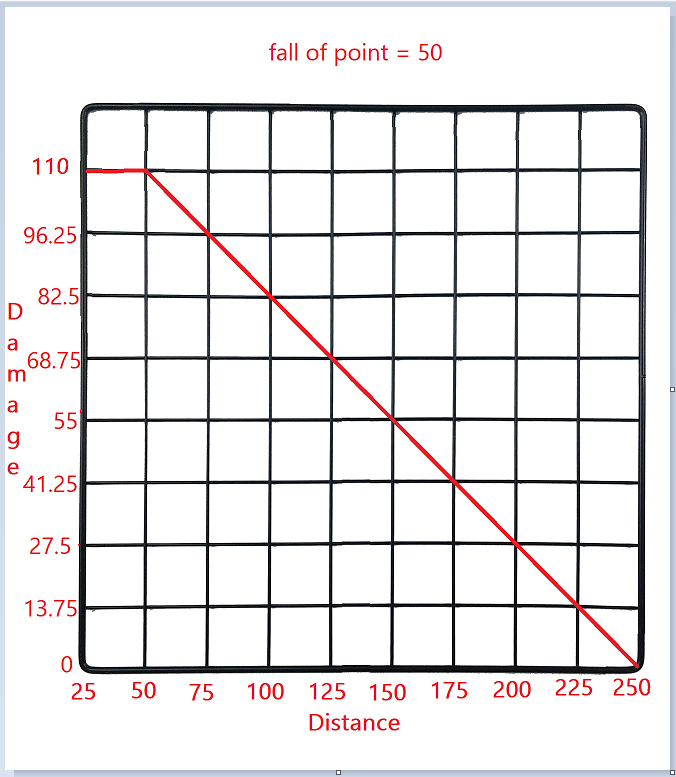# Calculating bullet damage with math (i suck at math)I’ve made a graph here which marks the amount of damage a gun should do at each range. What I need to figure out is how to find out what the damage would be

The damage will be 110 (max damage on the graph) if the player is less than 50 studs (fall off point) away from whoever is being shot

After 50 studs, I want the damage to decrease in a linear pattern until it reaches 0 damage (at 250 studs)

2 Likes

try a function like this to calculate the bullet damage

``````local function LineEquation(input, coord1, coord2)
local x1, y1 = table.unpack(coord1)
local x2, y2 = table.unpack(coord2)

local slope = (y2 - y1)/(x2 - x1)
local yIntercept = y1 - slope * x1

return slope * input + yIntercept
-- y = mx + b
end

local function CalculateBulletDamage(distance, damage, points)
local maxDamage, minDamage = table.unpack(damage)
local fallOffPoint, endPoint = table.unpack(points)

local calculatedDamage = LineEquation(distance, {fallOffPoint, maxDamage}, {endPoint, minDamage})
return math.clamp(calculatedDamage, minDamage, maxDamage)
-- clamp values over maxDamage and less than minDamage
end

print(CalculateBulletDamage(150, {110, 0}, {50, 250}))
-- 55
print(CalculateBulletDamage(79, {110, 0}, {50, 250}))
-- 94.05
print(CalculateBulletDamage(225, {110, 0}, {50, 250}))
-- ~13.75
print(CalculateBulletDamage(34, {110, 0}, {50, 250}))
-- 110
print(CalculateBulletDamage(300, {110, 0}, {50, 250}))
-- 0
``````
4 Likes

To people who could be referencing this post in the future, here is an image explanation of the math for the first print in @compacthq 's post:

Edit: First step would be to plot the two points on the grid, which are seen in this part of the script:

2 Likes